# NMTA Math: Rate of Change Chapter Exam

Exam Instructions:

Choose your answers to the questions and click 'Next' to see the next set of questions. You can skip questions if you would like and come back to them later with the yellow "Go To First Skipped Question" button. When you have completed the practice exam, a green submit button will appear. Click it to see your results. Good luck!

### Page 1

#### Question 1 1. The derivative of f(x) is 0 at how many points on the graph below?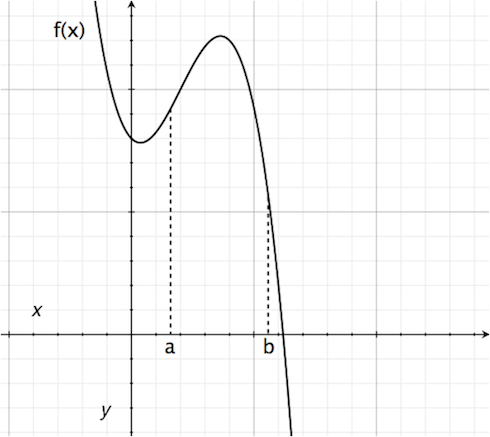#### Question 2 2. Consider the graph and question below..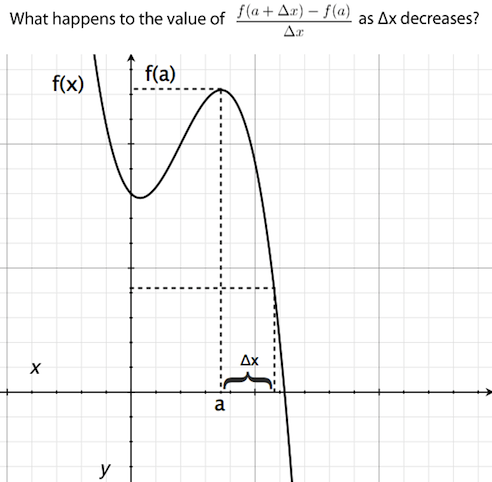#### Question 3 3. Consider the function f(x) given below. According to Rolle's theorem, between x=0 and x=pi, for how many values of x is the instantaneous rate of change equal to 0?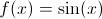#### Question 5 5. According to the mean value theorem, at how many points will the instantaneous rate of change equal the average rate of change between x = 1 and x = 3 on the following graph?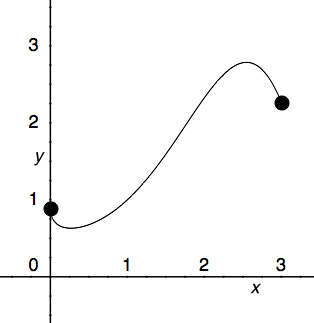### Page 2

#### Question 6 6. Where is f(x) differentiable?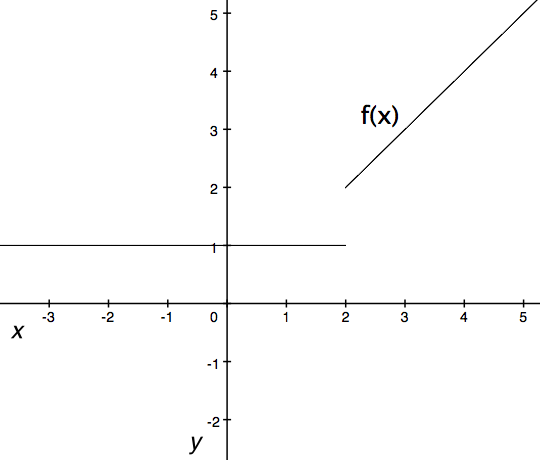#### Question 7 7. What is the average rate of change for the interval with endpoints A and B?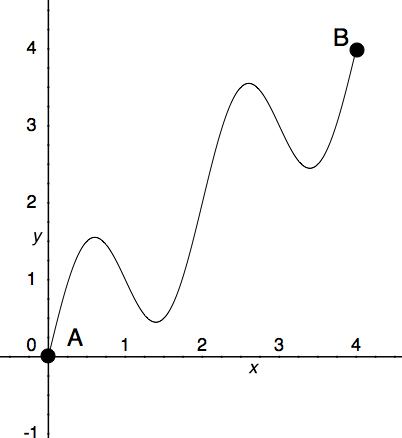#### Question 8 8. What is the value of f(x) at the red circle?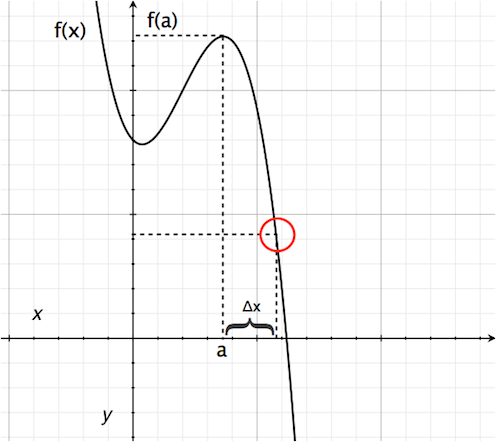#### Question 9 9. What is the rate of change at the point A in the graph below (for y in relation to x)?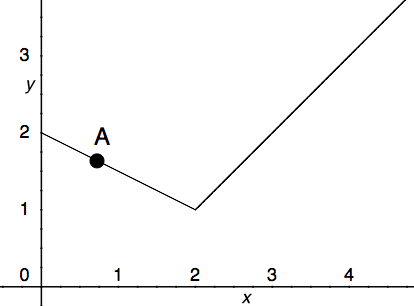### Page 3

#### Question 12 12. What is the rate of change at the point A in the graph below (for y in relation to x)?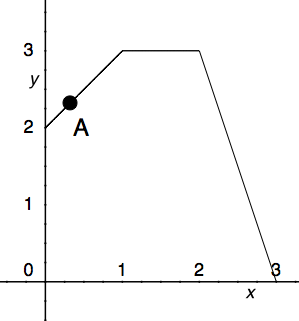#### Question 13 13. At how many points on the graph below is the derivative of f(x)=0?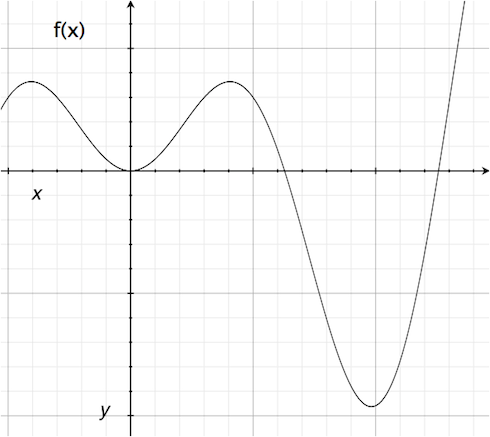#### Question 14 14. What is the rate of change at the point B in the graph below (for y in relation to x)? (In other words, what is the slope of the line from x = 2 to x = 3?)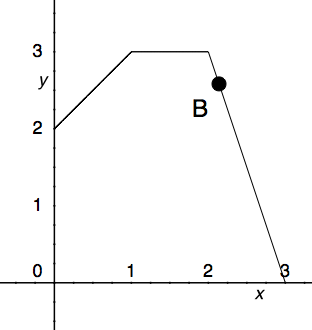#### Question 15 15. What is the rate of change at the point E in the graph below (for y in relation to x)?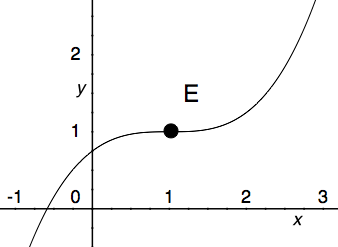### Page 4

#### Question 17 17. What is the derivative of f(t)?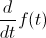#### Question 18 18. Where is f(x) differentiable?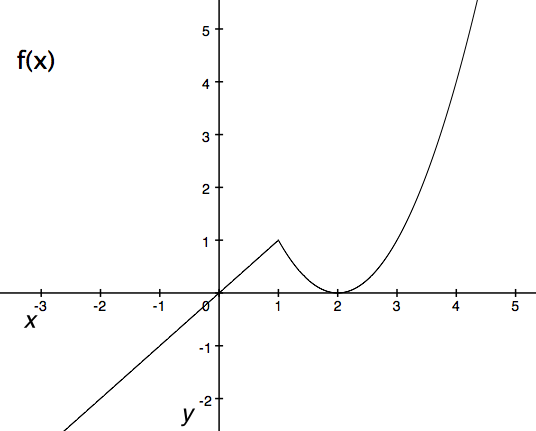#### Question 19 19. Consider the graph below. Between x=C and x=-C, for any value of C, will there be a point where the instantaneous rate of change is 0?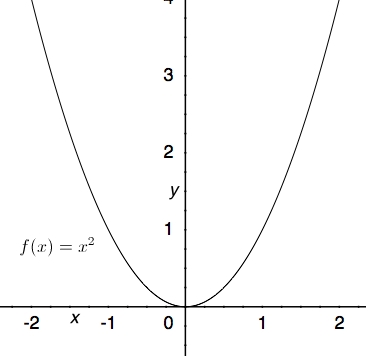#### Question 20 20. Where is f(x) differentiable?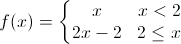### Page 5

#### Question 21 21. Which point on the following graph satisfies the mean value theorem between x = 0 and x = 3?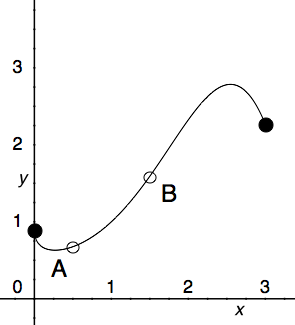#### Question 22 22. What is the rate of change at the point B in the graph below (for y in relation to x)?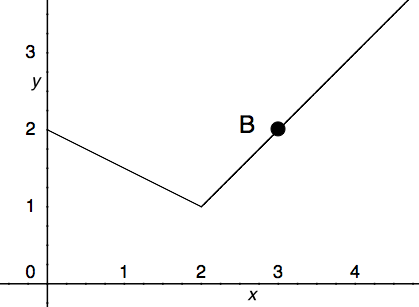#### Question 23 23. Where is f(x) differentiable?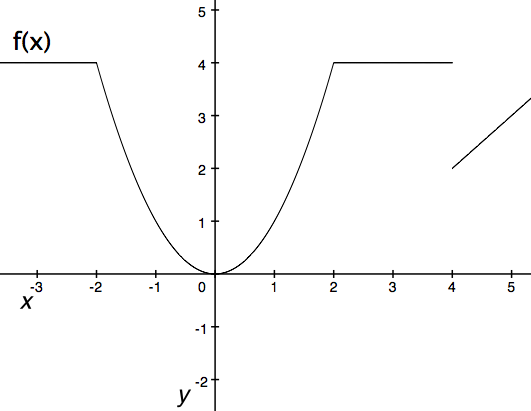#### Question 24 24. What is the approximate instantaneous rate of change at point C?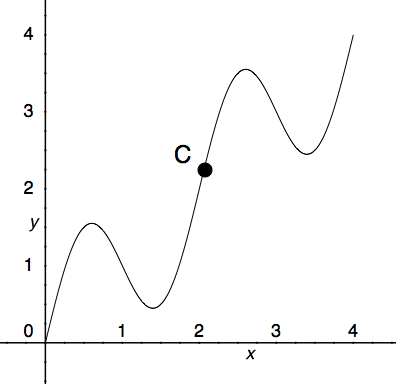#### Question 25 25. What is the derivative of the following?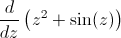### Page 6

#### Question 26 26. Where is y differentiable?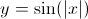#### Question 27 27. Consider the function f(x) given below. According to Rolle's theorem, for how many values of x is the instantaneous rate of change equal to 0?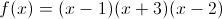#### Question 28 28. Which is greater, the derivative at a or the derivative at b?#### NMTA Math: Rate of Change Chapter Exam Instructions

Choose your answers to the questions and click 'Next' to see the next set of questions. You can skip questions if you would like and come back to them later with the yellow "Go To First Skipped Question" button. When you have completed the practice exam, a green submit button will appear. Click it to see your results. Good luck!

Support